## Q. 1. Why are solids rigid ?

Ans. At sufficiently low temperature, the termal energy is low and intermolecular ions forces between the particles (atomic lows or molecules) of a solid bring then so close that they cling to one another and occupy fixed positions. These can still oscillation to and from about their mean position. Thus solids are rigit.

Q. 2. Name one solid which shows both (i) Frenkel and Schottky defects. (ii) Frenkel and dislocation defects.

Ans. (i) AgBr (ii) ZnS.

Q. 3. What is Schottky and Frenkel defect.

Ans. (i) Schottky Defect : When a same number of cation and anion missing in latic there will number change in molecular formulae then this defect is known as schottky defect.
(ii) Frenkel Defect : In this defect some of cation change its interstitial (void) position with in a unit cell then there will no changing in molecular formulae as well as in density then there defect is known as frenkel defect.

Q. 4. What is semiconductor ? Describe the two main types of semiconductor and contrast their conduction mechanism.

Ans. Substances whose conductance lies between that of metals (conductors and insulators are called semi conductors.
Two main types of semi-conductors are n-type and p-type.
n-type semi-conductors : Group 14 elements like Ge, Si doped with group 15 elements such as P and As are called n-type semi-conductors. The symbol ‘n’ indicating that negative charge flows in them. The group 15 elements have one excess valence electron (compared to Si or Ge) after forming the four covalent bonds normally formed by group 14 elements. The excess electrons give rise to electrical conduction.

p-type semi-conductors : A group 13 element which has only three valence electrons forms an electron deficient bond or a hole. Such holes can move across the crystal giving rise to electrical conductivity. Thus impurity doped Si and Ge are semi-conductors whose conductivity increases with increasing temperature unlike metals whose conductivity decreases with increase in temperature. Group 14 elements doped with group 13 elements are called p-type seni-conductors. The symbol ‘p’ is used because in an electric field, the holes move through the crystal like positive charge, i.e., in a direction opposite to the flow of electrons.

Q. 5. Distinguish between :

(i) Hexagonal and monoclinic unit cells.
(ii) Face-centred and end-centred unit cells.
Ans. (i) Difference between Hexagonal and monoclinic unit cells are as follows :

 Hexagonal Unit cell Monoclinic unit cell (1) Axial distance or edge lengths a = b ≠ C a ≠ b ≠ C (2) Axial angles α = β = 90॰, α = γ = 90॰, β ≠ 90॰ (3) Examples γ = 120॰ Graphite, ZnO, CdS Monoclinic Sulphur, PbCrO2, Na2SO4, 10H2O

(ii) Difference between Face-centred and end centred unit cells are as follows :

 Face centred unit cell End centred unit cell It contains one particle present at the centre of its each face, besides the one that are at its corners. In this unit cell, one Constituent particle is present at the centre of any two opposite faces besides the one present at its corners.

Q. 6. (a) What is meant by the term ‘co-ordination number’ ?

(b) What is the co-ordination number of atoms :
(i) in a cubic close packed structure,
(ii) in a body centred cubic structure.

Ans. (a) Co-ordina- tion number : The total number of nearest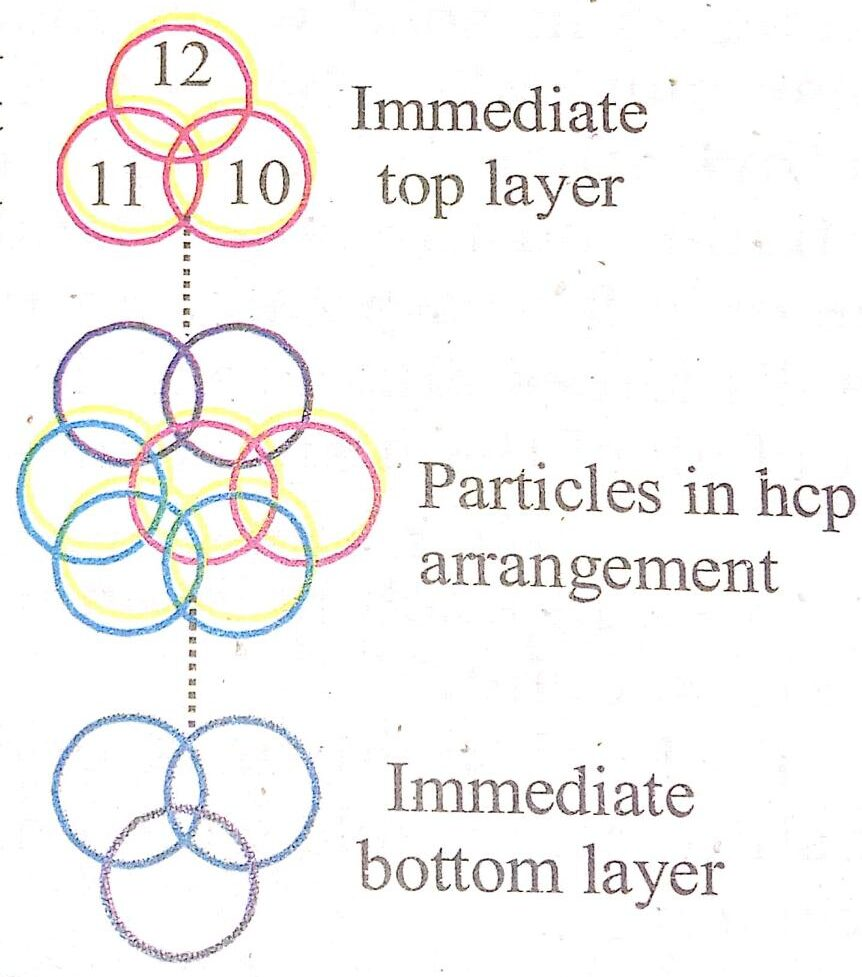Immediate neighbour atoms of a tion number : The total number of nearest neighbour atoms of a particular atom in a crystal lattice is known as its co ordination

number.Example :  In hexagonal close-packing (hcp) arrange-ment, each particle is surrounded by 12 equidistant spheres, i.e., 6 spheres in contact with in the same plane and 3 each in adjacent layers. One Representation of co-ordination immediately above and the number 12 in hcp packing other immediately below. This co-ordination number in help packing is 12.

(b) (i) 6 : 6, (ii) 8 : 8.

Q. 7. Define the term amorphous and crystalline solids with examples.

Ans. Amorphous Solids : A solid is said to be amorphous if the constituent particles are not arranged in any regular manner. They may have only short range order. Some examples of amorphous solids are glass, plastics, rubber etc.
Crystalline Solids : The substances whose constituents are arranged in a definite orderly arrangement are called crystalline solids. For example, NaCl, S, diamond, sugar etc. The crystalline substances have sharp melting points and have physical properties different in different directions, i.e., crystalline substances are Anistropic. They have long range and short range order.

Q. 8. What makes a glass different from a solid such as quartz ? Under what conditions could quartz be converted into glass ?

Ans. Quartz is a crystalline from of silica (SiO2). In it SiO4 tetrahedra are connected to one another in such a way that oxygen atom of each SiO4 tetrahedron is shared with another silicon atom. If quartz or SiO4 is melted and the melt is cooled, glass is formed which is amorphous. In glass SiO4 tetrahedra are randomly joined. It is super cooled liquid.

Q. 9. ‘Stability of a crystal is reflected in the magnitude of its melting points’.Comment. Collect melting points of solid water, ethyl alcohol, diethyl ether and methane from a data book. What can you say about the intermolecular forces between these molecules ?

Ans. Melting point of solid water = 273 K
M.Pt. of ethyl alcohol = 155.7 K
M.Pt, of diethyl ether = 156.8 K
M.Pt. of methane = 90.5 K

Higher the melting point of a crystal, greater are the forces holding the constituent particles (ions/atoms) together and hence greater is its stability.

The intermolecular forces in solid water (ice) and ethyl alcohal are mainly due to hydrogen bounding. Higher melting point of ice than ethyl alcohol shows that hydrogen bonding in ethyl alcohol inolecules is not as strong as in solid water molecules. Diethyl ether is a polar molecule. The intermolecular forces present in diethyl ether actually are dipole-dipole attractions. Methane, on the other hand is a non-polar molecule. The only forces present in them are the weak van der Waal’s forces and so melting point of methane is least out of all of them and is a gas at room temperature.

Q. 10. Give the significance of a lattice point.

Ans. (i) Each point in a lattice is called lattice site or lattice point.
(ii) Each point in a crystal lattice represents one constituent particle which may be an atom, a molecule or an ion.
(iii) Lattice points are joined by straight lines to bring out the gemetry of the lattice.

Q. 11. Name the prameters that characterise a unit cell.

Ans. A unit cell is characterised by the following parametrers :

(i) Its dimensions along the three edges a, band as shown. These edges may or may not be mutuall perpendicular.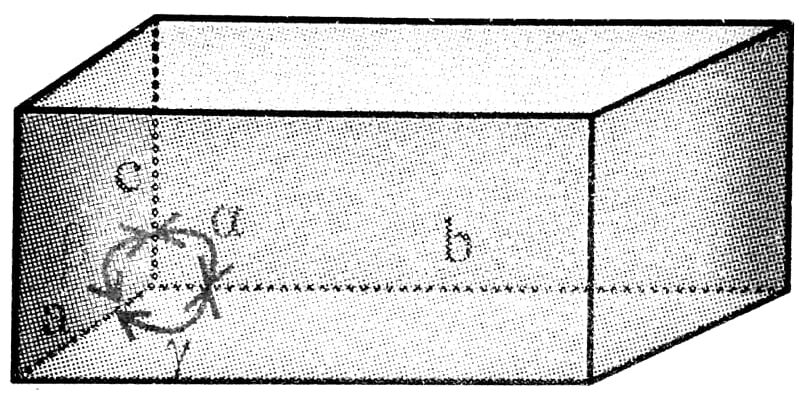.                                          Illustration of parameters of a unit cell
(ii) Angles between the edges α (between b and c),β (between a and c) and γ (between a and b).
Thus a unit cell is characterised by six parameters a, b, c and α, β,γ.

Q. 12. What type of stoichiometric defect is shown by (i) Zns, (ii) AgBr.

Ans. (i) Zns: It shows Frankel defect or dislocation defect. It is shown by ionic substances where there is a large difference in the size of the ions.

(ii) AgBr : It shows both Frenkel and Schottky defect.

Q. 13. Explain how vacancies are introduced in an ionic solid when a cation of higher valence is added as an impurity in it.

Ans. If molten NaCl containing a little amount of SrCl2 is crystallised, some of the sites of Na+ ions are occupied by Sr2+. Each Sr2+ replace two sodium ions to maintain electrical neutrality. It occupies the site of one Naion and the other site remains vacant. The cationic valances thus produced are equal in number to that of Sr2+ atoms.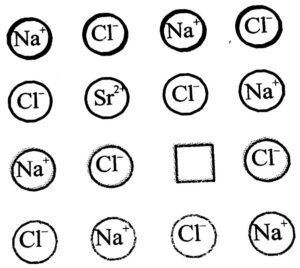Introduction of cation vacancy in Na+ Cl- by substitution of Na+ by Sr2+

Q. 14. Which out of Naci and CsCI, has larger coordination number ?

Ans. CsCl = 8; NaCl = 6, ∴  CsCl has larger coordination number.

Q. 15. Name one solid which shows both Frenkel and Schottky defects.

Ans. AgBr.

Q. 16. What happens to the structure of CsCl when it is heated to about 760 K?

Ans. CsCl structure is transformed of Naci structure.

Q. 17. Arrange simple cubic, body centred cubic, face-centred cubic and hexagonal close packing in the increasing order of the fraction of occupied space.

Ans. Simple cubic < bcc < fcc.

Q. 18. What is the effect of Schottky defects on the density of the crystal ?

Ans. The overall density of the crystal decreases.

Q. 19. Why is Frenkel defect not found in pure metal halides ?

Ans. Because in metal (alkali) halides, ions cannot get into interstital sites due to their large sizes.

Q. 20. In a close packing of N spheres, how many (i) tetrahedral and (ii) octachedral sites are present ?

Ans. (i) Tetrahedral = 2N (ii) Octahedral = N.

Q. 21. What is photovoltaic cell ?

Ans. Amorphous silicon acts as a typical photovoltaic cell in which as a result of sunshine (sunlight) electricity is produced.

Q. 22. How does temperature influence the conductivity of a cell ?

Ans. Its conductivity increases with increase in temperature.

Q. 23. What is the difference in semi-conductor obtained by doping silicon with As or with Ga ?

Ans. Si produced by doping with As is n-type semi conductor in which flow of current is due to electrons while silicon doped with Ga is p-type semi-conductor in which flow of current is due to positive holes.

Q. 24. Give three examples of amorphous solids.

Ans. Glass, rubber and plastics.

Q. 25. Why do solids have a definite volume ?

Ans. For a given mass of a solid, its density has a fixed value. Hence a solid has a definite volume. [Volume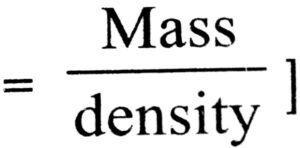Q. 26. What are interstitials in a crystals ?

Ans. Atoms or ions that fill the normal vacant interstitial voids in a crystal are called interstitials.

Q. 27. What is the effect of presence of Frenkel defects on the density of a crystal ?

Ans. The density of the crystal does not change.

Q. 28. How many atoms are present in the unit cell or (a) Simple cubic lattice, (b) BCC, (c) FCC ?

Ans. (a) 1, (b) 2 and (c) 4.

Q. 29. How can you convert NaCl structure into CsCl structure and vice-versa ?

Ans. NaCl structure can be converted into CsCl structure by application of pressure, while reverse can be done by heating CsCl to 760 K.

Q. 30. What is the coordination number of Na+ and C+ ions in NaCl structure ?

Ans. Six.

Q. 31. At what temperature ferrimagnetic substance changes to paramagnetic ?

Ans. 850 K

Q. 32. In a crystal of Zns, Zinc occupies tetrahedral voids. What is the coordination number of Zinc ?

Ans. 4

Q. 33. What is meant by superconductivity ?

Ans. At low temperature when a substance offers no resistance to the flow of electricity, the phenomenon is known as superconductivity.

Q. 34. What is energy gap in band theory ? compare its size in conductors, semi-conductor and insulators ?

Ans. Energy gap in band theory means the gap of energy between valence band and conduction band. The gap is in order conductor < semi-conductor < insulator.

Q. 35. What is F-centre ?

Ans. The site where electron is entrapped in the anion vacancy is called F-centre.

Q. 36. What is the maximum possible coordination number of an atom in an hcp crystal structure of an element ?

Ans. 12

Q. 37. What happens when ferrimagnetic Fe3O4 is heated to 850 K and Why ?

Ans. Ferrimagnetic Fe3O4 on heating to 850 K becomes Paramagnetic. This is due to greather alignment of spins in one direction on heating.

Q. 38. In CaF2 crystal, Ca2+ ions present in fcc arrangement Calculate the number of F-ions in the unit cell.

Ans. No. of Ca2+ ions per unit cell

= 8 ×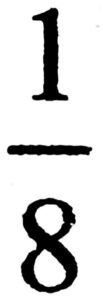+ 6 ×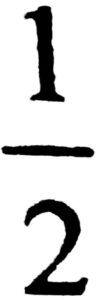= 4.

Hence, no. fo F-ions per unit cell = 2 x 4 = 8

Q. 39. What is the coordination number of an octahedral void ?

Ans. Six.

Q. 40. What type of crystal defect is produced when NaCl is doped with Mgcl2 ?

Ans. It is called impurity defect. A cation vacancy is produced. A substitutional solid solution is formed (because 2Na+ ions are replaced by one Mg2+ ions in the lattice).

Q. 41. Mention one property which is caused due to the presence of F-centre in a solid.

Ans. F-centre is responsible for the colour and paramagnetic behaviour.

Q. 42. At what temperature range most of the metals became super conductors ?

Ans. 2K – 5K

Q.43. How can a material be made amorphous ?

Ans. By melting the material and them cooling it studdenly.

## Class 12th Chemistry Short Type Question English

 S.N CHEMISTRY SHORT TYPE QUESTION 2022 1 SOLID STATE 2 SOLUTION 3 ELECTROCHEMISTRY 4 CHEMICAL KINETICS 5 SURFACE CHEMISTRY 6 GENERAL PRINCIPLES AND PROCESSES OF ISOLATION OF ELEMENTS 7 THE P-BLOCK ELEMENTS 8 THE D- AND f-BLOCK ELEMENTS 9 CO-ORDINATION COMPOUNDS 10 HALOALKANES AND HALOARENES 11 ALCOHOLS, PHENOLS AND ETHERS 12 ALDEHYDES, KETONES AND CARBOXYLIC ACIDS 13 AMINES 14 BIOMOLECULES 15 POLYMERS 16 CHEMISTRY IN EVERYDAY LIFE
You might also like